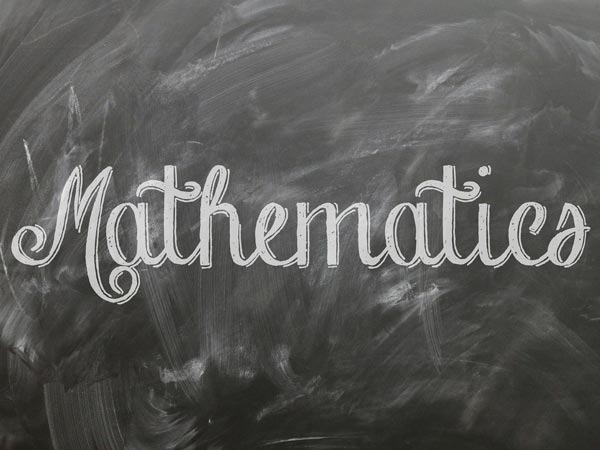# Important Topics To Revise In The CBSE Class 12 Mathematics Paper

By Sashikanth Sai

The CBSE Class 12 Mathematics paper will be held soon. This mega paper has a huge syllabus and travels along with you in most of the entrance examinations post the board exam. There is a chance of scoring 100 in this paper, however, a little attention and smart-study are required while preparing.

As the Mathematics paper is going to happen in less than a week, it is not a good idea to master every topic in the syllabus. We suggest students to go for a selective study. They should even go through the latest Mathematics sample paper issued by the CBSE, which will make them understand the pattern of the exam. As we said above that the smart-study is required for Mathematics paper, we take pleasure to list all the important topics unit wise.

## Unit-I – Relations And Functions

The types of relations, namely, reflexive symmetric, transitive and equivalence relations is very important. We can expect questions on the binary operations and composite functions. In the inverse trigonometric functions chapter, graphs and elementary properties are important.

## Unit-II – Algebra

The concept of notation, equality of matrix, types of matrices and identity matrix are important for 2 and 4 mark questions. The questions on transpose of a matrix, symmetric and skew symmetric matrices are also equally important. And never forget to revise the concepts on invertible matrices and proof of the uniqueness of inverse, if it exists.

In determinants chapter, determinant of a square matrix (up to 3 × 3 matrices) and properties of determinants are important. The consistency, inconsistency and number of solutions of system of linear equations are very important. Students should work on the solving system of linear equations in two or three variables (having unique solution) using inverse of a matrix.

## Unit-III – Calculus

Students should master concepts such as continuity & differentiability and Rolle's and Lagrange's mean value theorems. In the application of derivatives, increasing or decreasing functions, tangents and normals are important. One should know the basic concepts of integrals to understand the most important concept - applications in finding the area under simple curves.

The order and degree are important topics in the differential equations. The general and particular solutions of a differential equation and formation of differential equation whose general solution is given are very important topics.

## Unit-IV – Vectors and Three - Dimensional Geometry

The vectors and scalars concepts are very important for short and long answer questions. One should also study topics such as direction cosines and direction ratios of a vector and position vector of a point dividing a line segment in a given ratio. The properties and application of scalar (dot) product of vectors are also important.

Students should revise all the topics in the three dimensional geometry, however, give special attention to cartesian equation and vector equation of a line. They should focus on the angle between-two lines, two planes and a line and a plane besides distance of a point from a plane.

## Unit-V – Linear Programming

The mathematical formulation of linear programming problems is very important. The graphical method of solution for problems in two variables, feasible and infeasible regions (bounded and unbounded) are also the important topics.

## Unit-VI – Probability

Almost all the topics in the probability are important, however, one should give more importance to topics such as conditional probability, multiplication theorem on probability, Baye's theorem, repeated independent (Bernoulli) trials and binomial distribution.

These are some of the important topics we have identified based on the previous year question papers. Students are suggested to give priority to these topics during revision time. Wish you good luck!How To Score 100 In The CBSE Class 12 Mathematics Paper?

Subscribe Now

--Or--
Select a Field of Study
Select a Course
Select UPSC Exam
Select IBPS Exam
Select Entrance Exam